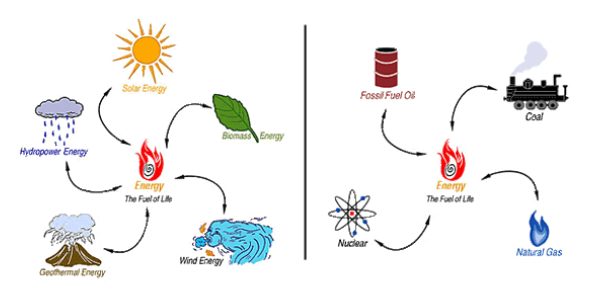# Energy Transformation Quiz: Trivia Questions!

14 Questions | Total Attempts: 11130SettingsDo you know anything about energy transformation? Would you like to take this quiz? You will have to understand what a change in composition or structure is called, how many different forms of energy are there, the law of conservation states, where the potential energy is the greatest, what occurs during energy transformations, and what transforms kinetic energy into electrical energy. This quiz is all about energy transformation. All the best.

• 1.
A change in composition or structure is called ____.
• A.

Energy

• B.

Transformation

• C.

Generator

• D.

Turbine

• E.

Reformation

• 2.
Energy forms can be ____.
• A.

Chemical

• B.

Thermal

• C.

Electrical

• D.

• E.

All of the above

• 3.
Energy is ___ changing.
• A.

Always

• B.

Never

• C.

Sometimes

• 4.
Energy transformations that involve chemical changes also produce ___.
• A.

Electricity

• B.

Friction

• C.

• D.

Heat

• E.

Sound

• 5.
The law of conservation states ___
• A.

Energy can be created and destroyed.

• B.

Energy is never created nor destroyed.

• C.

What goes up must come down.

• D.

Electrical energy can be transformed into other forms.

• 6.
_____ cars use an electric motor as well as a gasoline engine.
• A.

Electric

• B.

Mechanical

• C.

Hybrid

• D.

Generic

• E.

SUV

• 7.
Where is the potential energy greatest?
• A.

At the dip two cars past the red arrow.

• B.

As the car is moving up the hill 3 cars past the red arrow.

• C.

At the top of the hill before the red arrow.

• D.

There is no example of potential energy.

• 8.
Where is the kinetic energy GREATEST?
• A.

As the biker is climbing the hill.

• B.

As the biker is at the top of the hill.

• C.

AS the biker is on the downside of the hill.

• D.

There is no example of kinetic energy.

• 9.
__________ energy is the type of energy light has.
• A.

Potential

• B.

Thermal

• C.

• D.

Electrical

• 10.
During energy transformations, energy is never __________.
• A.

Released as heat

• B.

Used to increase an object's potential energy

• C.

Created or destroyed

• D.

Completely transformed

• 11.
A __________ is a device that transforms kinetic energy into electrical energy.
• A.

Furnace

• B.

Boiler

• C.

turbine

• D.

Generator

• 12.
A baseball is hit into the air with a bat. When does baseball have the greatest gravitational potential energy?
• A.

When it reaches its highest point

• B.

When the mechanical and kinetic energies of the baseball are equal

• C.

When it leaves the bat

• D.

When it hits the ground

• 13.
_________ is energy stored by things that stretch or compress.
• A.

Elastic potential energy

• B.

Chemical kinetic energy

• C.

Elastic kinetic energy

• D.

Gravitational potential

• 14.
What two factors determine how much gravitational potential energy is an object?
• A.

Weight and speed of the object

• B.

Shape of the object and its position relative to the ground

• C.

Weight of the object and height above the ground

• D.

Shape and speed of the object

Related TopicsBack to top